# Colleges with the highest SAT scores in Vermont

Top 7 colleges in Vermont with the highest SAT scores
Looking for the colleges with the highest SAT scores in Vermont? Well you're in luck! We've compiled a national college database and have created a list of the top 7 universities with the highest SAT scores in Vermont. These are the schools whose applicants had the highest average SAT scores in Vermont. And, since these tests are meant to determine academic prowess, they are arguably the schools with the most academically proficient students. You could even say these are the best colleges in Vermont. We also include each college's ACT scores and acceptance rate so that you can see where you would have the easiest or hardest time getting in. Read on to find out more.

## Middlebury College SAT scores

The average SAT score for Middlebury College is 1445.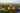The average SAT score of 1445 breaks down into:

• SAT math: 735

The average ACT score for Middlebury College is 33 and their acceptance rate is 15.4%.

## Bennington College SAT scores

The average SAT score for Bennington College is 1340.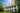The average SAT score of 1340 breaks down into:

• SAT math: 640

The average ACT score for Bennington College is 29 and their acceptance rate is 56.6%.

## University of Vermont SAT scores

The average SAT score for University of Vermont is 1270.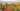The average SAT score of 1270 breaks down into:

• SAT math: 630

The average ACT score for University of Vermont is 29 and their acceptance rate is 67.3%.

## Saint Michael's College SAT scores

The average SAT score for Saint Michael's College is 1231.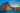The average SAT score of 1231 breaks down into:

• SAT math: 610

The average ACT score for Saint Michael's College is 27 and their acceptance rate is 85.1%.

## Champlain College SAT scores

The average SAT score for Champlain College is 1185.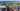The average SAT score of 1185 breaks down into:

• SAT math: 580

The average ACT score for Champlain College is 25 and their acceptance rate is 75.4%.

## Norwich University SAT scores

The average SAT score for Norwich University is 1130.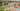The average SAT score of 1130 breaks down into:

• SAT math: 560

The average ACT score for Norwich University is 23 and their acceptance rate is 65.7%.

## Castleton State College of Vermont SAT scores

The average SAT score for Castleton State College of Vermont is 1040.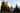The average SAT score of 1040 breaks down into:

• SAT math: 510